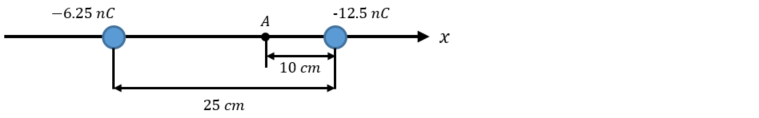# Problem: Two point charges having charge of -6.25 x 10 -9 C and -12.5 x 10 -9 are 25.0 cm apart. a) Calculate the magnitude of Coulomb force between them. Is it repulsive or attractive? b) What is the magnitude of electric field at point A? What is its direction?

🤓 Based on our data, we think this question is relevant for Professor Efthimiou's class at UCF.

###### Problem Details

Two point charges having charge of -6.25 x 10 -9 C and -12.5 x 10 -9 are 25.0 cm apart.

a) Calculate the magnitude of Coulomb force between them. Is it repulsive or attractive?

b) What is the magnitude of electric field at point A? What is its direction?What scientific concept do you need to know in order to solve this problem?

Our tutors have indicated that to solve this problem you will need to apply the Electric Field concept. You can view video lessons to learn Electric Field. Or if you need more Electric Field practice, you can also practice Electric Field practice problems.

What is the difficulty of this problem?

Our tutors rated the difficulty ofTwo point charges having charge of -6.25 x 10 -9 C and -12.5...as low difficulty.

How long does this problem take to solve?

Our expert Physics tutor, Douglas took 9 minutes and 5 seconds to solve this problem. You can follow their steps in the video explanation above.

What professor is this problem relevant for?

Based on our data, we think this problem is relevant for Professor Efthimiou's class at UCF.